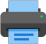Lista de exercícios do ensino médio para impressãoSelect all.
print selected exercises
The length of the two parallel sides of a right trapezoid are 6 cm and 8 cm, respectively top and bottom bases, and the altitude is 4 cm. The distance between the intersection of the two straight lines that contain the non-parallel legs and the midpoint of the major base is:
a)
$\,5\sqrt{15}\,$ cm
b)
$\,2\sqrt{19}\,$ cm
c)
$\,3\sqrt{21}\,$ cm
d)
$\,4\sqrt{17}\,$ cm
e)
none of these